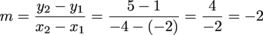Study Guide

# Writing Equations of Lines

## Writing Equations of Lines

We've been called to the scene of a crime. The cops are looking for the equations of their top suspects, but there is limited evidence at the scene. Luckily, Shmoop has some tricks for tracking down equations of lines. Depending on what we already know, we have three ways to write the equation of a line.

y = mx + b

If we know the slope, m, and the y-intercept, b, we can go straight to slope-intercept form and solve the case in record time.

What happens when we have some random point on the line, instead of the y-intercept specifically? Then we can find the point-slope form of the equation using, yes, the point and the slope. Here's what point-slope form looks like:

yy1 = m(xx1)

Now let's see it in action.

### Sample Problem

Find the equation of the line that passes through (-4, 1) and is parallel to 3x – 3y = 9.

Well, we have a point, but not the slope. It's a good thing that parallel lines share so much, including their clothes, their taste in food, and their slopes. We'll just rearrange the given equation into slope-intercept form to find it.

3y = 3x – 9

y = x – 3

We have a slope, m = 1. Now use the point-slope method to get the equation we're really after.

yy1 = m(xx1)

Here's our formula. The values x1 and y1 come from our point, (x1, y1). Just like you'd expect, x1 goes with x, and y1 goes with y. Plug in the slope and the point (-4, 1) to see what's up.

y – 1 = 1(x + 4)

Notice that y1 and x1 are subtracted from y and x in the formula, so their signs will be flipped-turned upside down from usual.

We're going to put the equation into slope-intercept form at this point. While the point-slope form is okay, it's a little derivative. And no, this isn't a Calculus pun—this time.

y – 1 = 1(x + 4)
y = x + 5

If we pick two different points on a line, we'll have two different point-slope equations, but they will simplify into the same, unique slope-intercept equation. One is a unique special snowflake, while the other is a shape-shifting chameleon…snowflake.

We may not have thought through that analogy enough. Moving on.

Our final technique is to be used on the toughest of cases, when we don't even have the slope of the line we're searching for. The two-points method only requires that we have two points from the line, (x1, y1) and (x2, y2). A big magnifying glass helps with the whole detective image we have going here, but it isn't necessary.

### Sample Problem

Find the equation of the line that passes through (-2, 1) and (-4, 5).

The first step of the two-points method is to find the slope. We can do that; we know the formula, we have the power.Finding the slope is the two-points method's only contribution to the problem. From here we use the point-slope method to find the equation of the line. Hopefully we won't find more than we bargained for, like an ancient mummy's curse or a bear.

yy1 = m(xx1)

y – 1 = -2(x – (-2))

y = -2x – 3

We have our choice of which point to use, so we used the one with smaller numbers. We like small, easy-to-use numbers.

Anyway, the problem is done and our bodies aren't covered in boils or scratch marks, so we think we're in the clear.

### Summary

Which detective technique should be used on any given problem? Here's the breakdown.

• If we have the slope and the y-intercept, we're done already. Just plug them into the slope-intercept form.
• y = mx + b
• If we have the slope and one point from the line, use the point-slope method, then simplify it to slope-intercept form.
• yy1 = m(xx1)
• If we don't have the slope, but we do have two points from the line, calculate the slope, pick one of the points, and go to town with the point-slope method.

We stepped in and, using our newly acquired math expertise, solved all the mysteries using what the cops thought was insufficient evidence. Not too bad for our first day on the force.